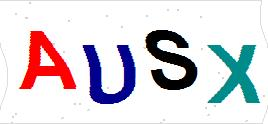# 静电电阻测试仪

]资料

## 静电电阻测试仪的详细介绍

TEST）按钮，持续发亮的发光二极管（LED）即指示出测量的表面阻抗量级。
103=1

104=10

105=100

106=1

107=10

108=100

109=1000

1010=10000

1011=100000

1012=1000000

>1012=点击换一张

2.如有必要,请您留下您的详细联系方式!

## 静电电阻测试仪 的相关产品